All submissions of the EM system will be redirected to Online Manuscript Submission System. Authors are requested to submit articles directly to Online Manuscript Submission System of respective journal.

Gravitation

Ferent AI*

Augustin Maior College, Cluj-Napoca, Romania

*Corresponding Author:
Augustin Maior College, Cluj- Napoca, Romania
Tel: 40264 - 546 244
E-mail:
[email protected]

Received date: 27/08/2015 Accepted date: 27/09/2015 Published date: 29/09/2015

Visit for more related articles at Research & Reviews: Journal of Pure and Applied Physics

Abstract

The Photon – Graviton pair (coupled) has the same speed and frequency, and the photon energy divided by the graviton energy is the electromagnetic energy divided by the gravitational energy, the electromagnetic force (for virtual photons) divided by the gravitational force (for virtual gravitons). This helped me to go beyond the Planck wall. It is our ultimate task to discover a new quantum theory which breaks the wall of Planck scale and creates a new frontier. I found another wall, the Ferent wall beyond the Planck wall, where the Planck constant h=6.62606957×10-34 J· s is replaced by Ferent constant a = 1.590511178×10-76 J· s. I replaced Max Planck equation E = h × f with the Ferent equation for the energy of a photon: E = h × f + a × f I discovered the impulse of the graviton: p = a / λ and replaced Heisenberg Uncertainty Principle Δp × Δx ≥ h / 4π with Ferent Uncertainty Principle: Δp × Δx ≥ a I discovered a new electromagnetic theory. My gravitation theory explains dark matter and dark energy! The graviton has negative impulse, negative mass and negative energy. I am the first who understood and explained that the gravitons with the speed of light, c = 2.9979 × 108 m/s, are too slow to keep the constellations and the galaxy together. I explained the gravitation with high speed gravitons, va = 1.001762 × 1017 m/s. The mass of a body, system M = m1 + im2 where m1 is the real mass and m2 is the imaginary mass, is the rest mass of the gravitons. I calculated the volume of the universe at Planck wall, VP = 5.819 × 10-45 m3 and the volume of the universe at Ferent wall, VF = 3.355 × 10-130 m3 My quantum gravity theory shows that the gravitons are too small to be detected by today’s technology.

Keywords

Decoherence, Electromagnetic, Gravitation, Frequency, Electrons, Sinusoids, Gravitational.

Introduction

All the gravitation theories are limited to the speed of light. In these theories the speed of gravitations (gravitons) is equal with the speed of light. In my view the perturbation done by the photon in a gravitational field is equal with one graviton. Because light has gravitational property, light is deviated by mass; from here author started a new electromagnetic theory. So dechoerence is due to the gravitational field, to the gravitational waves generated by the observer. The electromagnetic force divided by the gravitational force is the photon energy divided by the graviton energy. This brought me to another wall beyond the Planck wall, with a length smaller than the Planck length. How about the graviton speed? I realized that the speed of light is too small for the graviton speed. If the graviton has only the speed of light, because the black hole escape velocity is higher than the speed of light, my conclusion was: the graviton speed is much higher than the speed of light. Gravitational fields are conservative; the work done by gravity from one position to another is path-independent.

Theory

The oscillation of an electron emit, radiate to space a photon and a coupled graviton, with the same speed, the speed of light and the same frequency. The energy E contained in a graviton, which represents the smallest possible 'packet' of energy in a gravitational wave, is the ‘a’ the Ferent constant times the frequency

The energy E of a photon

Planck discovered that physical action could not take on any indiscriminate value. Instead, the action must be some multiple of a very small quantity called Planck constant. The Planck constant is a physical constant that is the quantum of action, describing the relationship between energy and frequency. The energy E contained in a photon, which represents the smallest possible 'packet' of energy in an electromagnetic wave, is Planck’s constant times the frequency:

E = Planck’s constant × frequency.

E = h × f

Max Planck equation for the energy of a photon E = h × f is incorrect because does not contains the energy of the graviton, because light has gravitation!

Ferent equation for the energy of a photon: E = h × f + a × f

Where h – is the Planck constant h = 6.62606957×10-34J·s and a - is the Ferent constant a = 1.590511178×10-76 J·s

The electromagnetic field is a physical field that is produced by electrically charged objects. However, the gravitational force is extremely weak when compared to the electromagnetic force. In fact it is only about 1/s of the strength of the electromagnetic force.

s = 4.166 × 1042

Light is part of a group of phenomena collectively known as electromagnetic radiation. All members of the group can be created by accelerating electric charges, usually electrons. Radiation occurs whenever electric charge is accelerated. Radio and television signals are caused by electrons accelerating in radio antennas. The electric and gravitational fields are quite similar. Electrons are the smallest parts of the atom, they have the smallest mass. The oscillation of an electron emit, radiate to space a photon. Therefore photons have gravity, because they have relativistic mass.

Electromagnetic theory

In a view electromagnetic wave is the superposition of 3 sinusoids, because the photon has relativistic mass and travels with a coupled graviton:

The Photon - Graviton pair (coupled) has the same speed and frequency and the solutions of Ferent electromagnetic equation:

E (r, t) = E0 cos (ωt – kr +ϕ0)

B (r, t) = B0 cos (ωt – kr +ϕ0)

g (r, t) = E0 cos (ωt – kr +ϕ0)

This means the Ferent electromagnetic equation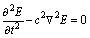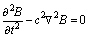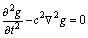My equations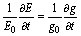A changing electric field generates a changing gravitational field and both sinusoids have the same derivative divided by their amplitude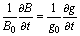A changing magnetic field generates a changing gravitational field and both sinusoids have the same derivative divided by their amplitude

Decoherence explained by my theory

The electromagnetic wave is the superposition of 3 sinusoids; this means the electromagnetic wave will be collapsed by the presence of an electric field, of a magnetic field, of a gravitational field, by another electromagnetic wave. In my electromagnetic theory, gravity does collapse quantum superpositions, gravity bends light because light has 3 sinusoids, has a gravitational sinusoid! In Maxwell electromagnetic theory, gravity does not collapse quantum superpositions, gravity does not bend light, because light has only 2 sinusoids! So decoherence is due to the gravitational field, for example to the gravitational waves generated by the observer in double-slit experiment.

Field

Field is a physics term for a region that is under the influence of some force that can act on matter within that region. The electromagnetic field is mediated by the exchange of virtual photons. The gravitational field is mediated by the exchange of virtual gravitons. For example, the Sun produces a gravitational field that attracts the planets in the solar system and thus influences their orbits.

The Ratios

The magnetic force always acts at right angles to the motion of a charge, it can only turn the charge, it cannot do work on the charge. The strength s, of the electromagnetic force relative to gravity force: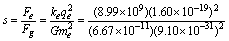s = 4.166 × 1042

With s I calculated the Ferent constant.

Gravitation

Gravitation is a phenomenon by which all things attract one another including sub-atomic particles, planets, stars and galaxies. The four fundamental forces: the gravitational force, the electromagnetic force, the strong nuclear force and the weak nuclear force, are the four fundamental forces. The gravitational force is mediated by a massless particle called the graviton. Because gravitation is an inverse square force of apparently infinite range it can be implied that the rest mass of the graviton is zero. Gravitational fields are conservative; the work done by gravity from one position to another is path-independent.

The ‘a’ constant

The electric and gravitational fields are quite similar. In my view, the energy E contained in a graviton, which represents the smallest possible 'packet' of energy in an gravitational wave, is the ‘a’ constant times the frequency

E = a × f

Now I consider the case of a photon (virtual photon) and of a coupled graviton (virtual graviton) with the same frequency and the same speed emitted by an electron. Because the strength, of the electromagnetic energy relative to gravity energy it is the strength s = 4.166 × 1042, the photon energy Ep = h × f, divided by the graviton energy Eg = a × f it is s = 4.166 × 1042

The value of ‘a’

We have s = h / a

Adrian Ferent constant a = h / s

The Planck constant h=6.62606957×10-34 J·s

This means the Ferent constant

a = 1.590511178×10-76 J· s

The impulse of the graviton

The impulse of a photon: p = h / λ

The impulse of the graviton: p = a / λ

I derived this equation from the Klein–Gordon equation.

Because the graviton has the rest mass equal to zero and the speed is equal to the speed of light E = p × c

E = a × f from here p × c = a × f, and because f / c = λ, we have:

The impulse of the graviton:

p = a / λ

That is why the gravitons are too small to be detected by today’s technology.

The Uncertainty Principle

Heisenberg Uncertainty Principle is limited for gravitons!

Δp × Δx ≥ h / 4π

Ferent Uncertainty Principle:

Δp × Δx ≥ a / 4π

From this equation: p = a / λ by substituting Δx for λ :

Δp × Δx = a this means Δp × Δx ≥ a, much stronger inequality than the Heisenberg uncertainty principle.

This means Δp × Δx > a / 4π and in the same way like in the case of Heisenberg

Uncertainty Principle, this equation can be refined to Δp × Δx ≥ a / 4π

The energy of the graviton

Because E = a × f and p × c = a × f ; p = m × c ; m – the relativistic mass of the graviton. The energy of the graviton: E = m × c2

The Ferent length

Using the same equations as for the Planck units I can calculate all the units at Ferent wall.

Gravitational constant G = 6.67384×10-11m3 ·kg-1 ·s-2

The Ferent length la =7.918359×10-57 meters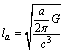The Ferent wall

I found another wall the Ferent wall beyond the Planck wall, where the Planck constant h=6.62606957×10-34 J· s is replaced by Ferent constant a = 1.590511178×10-76 J· s.

I replaced Max Planck equation E = h × f with the Ferent equation for the energy of a photon: E = h × f + a × f

I discovered the impulse of the graviton: p = a / λ

I replaced Heisenberg Uncertainty Principle Δp × Δx ≥ h / 4π with Ferent Uncertainty

Principle: Δp × Δx ≥ a

The energy of the graviton: E = m × c2

The Ferent length la = 7.918359×10-57 meters

discovered a new electromagnetic theory. At Ferent wall emerged the gravitation and the gravitons with the energy Eg = a × f and the speed equal with the speed of light. Now I can calculate using the same equations from the Planck wall for the Planck units, the Ferent length, the Ferent time, the Ferent energy…I can define very well the Ferent wall! During the Big Bang first emerged the gravitational force at Ferent wall and after that the electromagnetic force, the strong nuclear force and the weak nuclear force. This means I discovered a new gravitation theory and I found how the gravitation and the gravitons emerged at Ferent wall! I can stop here with my gravitation theory. Why to go beyond, what I considered it is limited is the speed of the gravitons equal with the speed of light. Why gravitons faster than the speed of light?

Faster than speed of light!

I am the first in the world who understood and explained that the gravitons with the speed of light are too slow to keep the constellations together. The gravitons with the speed of light are too slow for the escape velocity of a black hole. The black hole escape velocity exceeds that of light.

Big Bang

Bang My theory is the same if the universe did not start with the Big Bang. My gravitation theory explains what happened at Ferent wall and after the expansion of the Universe attains the Ferent wall .

Two important walls:

The Ferent wall: here at time t = 1.294 × 10-86 s were created Ferent matter and gravitons with the speed of the gravitons va = 1.001762 × 1017 m/s.

The Planck wall: here at time t = 5.391 × 10-44 s were created matter and photons, with the speed of the photons c = 2.997924 × 108 m/s.

Einstein field equations

The Einstein (Hilbert) field equations can be interpreted as a set of equations dictating how matter/energy determines the curvature of spacetime. Einstein reinterpreted the gravity not as a force pulling on objects but as a curvature of spacetime. For Einstein objects falling in a gravitational field like around the Earth aren't being pulled but are simply moving along geodesics in the warped spacetime surrounding the Earth. For Einstein, the ball falls because spacetime is curving, not because there is a force pulling it back to Earth! Einstein was erroneous, because in my view, the gravitons, the virtual gravitons with high speed va moving in the gravitational field are the force carriers!

Discrete space

Today we know that the space is not an infinitely divisible continuum, it is not smooth but granular and Planck length and Planck volume gives the size of its smallest possible grains.

Planck length, lP = 1.616199×10-35 m

Planck volume, lP3 = 4.22419×10-105 m3

Conservation of Energy

The law of conservation of energy states that the total energy of an isolated system cannot change in time! The Planck energy is nature’s maximum allowed matter energy for point-energy (quanta). During the Big Bang, this means the Ferent energy, at Ferent wall, is equal with the Planck energy. From here I calculated the speed of a free (not coupled) graviton!

The speed of the graviton

The speed of the graviton Va, where a=a/2π

Gravitational constant G = 6.67384×10-11m3 ·kg-1 ·s-2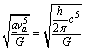From this equation the speed of the graviton is va = 1.001762 × 1017 m/s much faster than the speed of light!

Ferent volume

Ferent length: lF = 1.296 × 10-69 m

Ferent volume lF 3 = 2.18 × 10-207 m3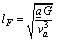Ferent time

Ferent time tF = IF / Va = 1.294 × 10-86 s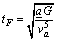Ferent frequency

Ferent frequency fF = 1 / tF = 7.728 × 1085 Hz

Ferent energy

Ferent energy EF = a × fF = 1.956 × 109 J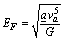Ferent mass

Ferent mass mF = EF / Va2 = 1.949 × 10-25 kg

Ferent density

Ferent density ρF = mF / lF3 = 8.94 × 10181 kg/m3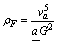The volume of the universe

Mass of the universe: M = 3 × 1052 kg

The volume of the universe at Planck wall:

VP = M / ρP = 3 × 1052 kg / 5.155 × 1096 kg/m3 = 5.819 × 10-45 m3

The volume of the universe at Ferent wall:

VF = M / ρF = 3 × 1052 kg / 8.94 × 10181 kg/m3 = 3.355 × 10-130 m3

This means between Ferent wall and Planck wall the universe expanded VP / VF = 1.546 × 1085 times in a period t = tP - tF = 5.391 × 10-44 s.

Dark matter

At Ferent wall due to the extraordinarily small scale of the universe at that time, gravitation was the only physical interaction. At Ferent wall were created Ferent matter and gravitons. What is Ferent matter?

Ferent density ≥ Ferent matter density > Planck density

8.94 × 10181 kg/m3 ≥ Ferent matter density > 5.155 × 1096 kg/m3

That is why Ferent matter particles do not carry any electric charge. Only a small percentage of Ferent matter becomes matter at Planck wall. Ferent matter is 84.5% of the total matter in the universe. The majority of Ferent matter is the core of the supermassive black hole, in the center of each galaxy. Ferent matter plays a central role in galaxy formation and evolution. At the largest-size scales dark matter dominates the dynamics of galaxy clusters and superclusters. How you can see the properties of Ferent matter are the properties of dark matter! Dark matter is similar to Ferent matter, interacts only gravitationally. My gravitation theory explains dark matter!

Proposed quantum gravity theories: String theory, LQG

There have been numerous theories of gravitation since ancient times. The graviton in String theory is a closed string with the length of couple Planck lengths. This means the graviton is gigantic that is why the String theory is limited to the speed of light! LQG: the predicted size of this structure is the Planck length. According to this theory, there is no meaning to distance at scales smaller than the Planck scale. My quantum gravity theory breaks the wall of Planck scale. Anyway there are numerous quantum gravity theories, but all of them are limited to the speed of light .

Quantum gravity theory and information

In my view the information of our universe is in the gravitational field carried by gravitons (qubits). Only a small part of the information is carried by photons. The gravitons were not detected because they have a very small energy; the gravitons are too small to be detected by today’s technology. The light cannot escape from the black hole, but the small gravitons with high speed and high frequency can very easy go through the event horizon.

Quantum entanglement explained

Quantum entanglement: explains that information moves faster than light. If we have two electrons close together, they can vibrate in unison, entangled electrons, according to quantum theory. If we then separate them an invisible cord emerges and connects the two electrons, even though they may be separated by many light years. If we jiggle one electron, the other electron ‘senses’ this vibration faster than the speed of light. Einstein named ‘spooky action at a distance’; he thought that this contradicts the quantum theory, since nothing can go faster than light. In my view when the electron is jiggled it is a change in the gravitational field and the gravitons with a speed Va = 1.0017620 ×1017 m/s faster than the speed of light will change the state of the entangled electron.

Gravitons with negative impulse, negative mass and negative energy

At Ferent wall started our Universe: matter has positive energy and the gravitational field has negative energy. If the two values cancel out, the universe has zero energy and can theoretically last forever. The negative energy (- E) is needed to offset the positive energy +E of matters, negative gravitational potential energy offset positive energy.

E = 0 = +E + (- E)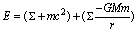The gravitons give a negative impulse to mass-carrying particles to attract them! This means the impulse of the graviton is negative p = - m × v and I calculated the speed of the graviton va. Because the impulse is negative, the relativistic mass - m of the graviton is negative! If the relativistic mass m of the graviton is negative, this imply that the energy of the graviton is negative, E = - m × v2!

Gravitational fields are conservative; the work done by gravity from one position to another is path-independent.

Conservative vector field is a vector field that is the gradient of a scalar potential V(r).

Gravitational field: g(r) = - ∇V(r)

Conservative vector fields have the property that the line integral is path independent, this means the choice of integration path between any point and another does not change the result.

Negative mass

Negative mass possess this property, such as accelerating in the direction opposite of applied force. Negative mass is mathematically consistent and introduces no violation of conservation of momentum or energy. Newton's law of universal gravitation states that any two bodies both with positive mass or both with negative mass in the universe attract each other. But in the case of both bodies having negative mass the motion will be repulsive. For two gravitons the equation: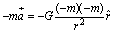Two objects with negative mass would accelerate away from each other, they repel each other. This means the gravitons repel each other because they have negative mass. Another case: a negative mass (energy) less massive (talking about absolute values here) than a positive mass body, is accelerated in the direction of positive mass body and it would move much faster and will ‘catch up’ with the positive mass body (attractive effect). Positive mass has attractive effect on each other so it forms planets, stars and galaxies. Negative mass has repulsive effect on each other so it cannot form planets, stars and galaxies. Positive mass– Ferent matter and negative mass-gravitons emerged together at Ferent wall in the energy and momentum conservation state. Negative masses because of repulsive effect are uniformly distributed in space. Uniformly distributed negative mass receive attractive effect from massive positive mass, this is gravitation. The expansion of the universe takes place because of negative mass repulsive effect.

Conservation of impulse and conservation of energy

My theory is completely mathematically consistent and introduces no violation of conservation of momentum or energy. We have two masses equal in magnitude but opposite in sign, and then the momentum of the system remains zero if they both travel together and accelerate together, no matter what their speed:

Conservation of momentum:

P = m× v + (- m) × v = [m + (- m)] × v = 0 × v = 0

E = m× v2 × 1/2 + (- m) × v2 × 1/2 = [m + (- m)] × v2 × 1/2 = 0 × v2 × 1/2 = 0

We have positive mass m, the anti-gravitons and the negative mass –m, the gravitons at Ferent wall. Ferent matter contains the anti-gravitons. Positive mass has attractive effect on each other so it forms dark matter and matter at Planck wall. This means Ferent matter at Planck wall was divided in dark matter and matter. Because the Ferent matter the positive mass cannot have the speed of the gravitons, between the Ferent wall and the Planck wall the universe had a negative acceleration: (c - va) / (tP - tF) = ( - 1.001762 × 1017 m/s) / 5.391 × 10-44 s = - 1.858 × 1060 m/s2.

The others gravity theories do not explain why the universe expanded and why the universe slowed down.

Mass equation

Negative energy and positive energy emerged together at Ferent wall. Negative energy generated negative mass - gravitons and positive energy generated positive mass - Ferent matter. Newton’s law of motion explains that positive mass formed massive positive mass structure and at the Planck wall formed planets, stars and galaxies. The mass of a body M = m1 + im2 where m1 is the real mass and m2 is the imaginary mass, is the rest mass of the gravitons. Since the relativistic mass of the gravitons is negative imply that the rest mass m2 is imaginary.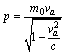The denominator is imaginary va > c, this imply that the rest mass of the gravitons m0 must be imaginary; because the impulse p is an observable and must be real. For an isolated system, body the mass M, the mass m1 the real mass and m2 the imaginary mass are the same, this means the mass of gravitons is constant. The real mass m1 is much bigger than m2 the imaginary mass.

It is like the difference between Panck mass mP = 2.176 × 10-8 and Ferent mass mF = 1.949 × 10-25 kg.

Dark energy

Gravitons traveling between galaxies are dark energy. These gravitons repel each other. The negative mass, the gravitons are the dark energy that accelerates in the direction of cosmic expansion. Repulsion is required for cosmic expansion. The even distribution of gravitons means that dark energy does not have any local gravitational effects, but rather a global effect on the universe as a whole.

Proof for my theory

When people saw the same constellations for years, they were supposed to understand that the gravitons with the speed of light are to slow to keep those stars together. That is why Einstein-Hilbert gravitational field equations, String theory, LQG… are limited, because are limited to the speed of light. A graviton or a virtual graviton needs less than one second to travel between stars located within 10 light-years. A light year value is ly = 9.460536207×1015 m, the speed of the graviton va = 1.001762 × 1017 m/s and t = 10 × ly / va, this means t < 1s.

Another proof for my theory

There are galaxies 11 billion light years away, which are receding from us. Why? Because in my view the speed of the graviton va is too small to keep the galaxies together.

Another proof for my theory

At the event horizon of the black hole from our galaxy the Gravity = 0, because the escape velocity is greater than c - speed of light and by Einstein gravitation theory, String theory, LQG...the gravitons have the speed of light. This means the black hole do not attract anything! The gravitons need the speed va to go through the event horizon.

Conclusions

consider the theory of gravity the most important theory because since ancient times the brightest people tried to explain the most common force in nature and how the universe began. I found another wall the Ferent wall beyond the Planck wall, where the Planck constant h=6.62606957×10-34 J· s is replaced by Ferent constant a = 1.590511178×10-76 J· s.

I replaced Max Planck equation E = h × f with the Ferent equation for the energy of a photon:

E = h × f + a × f

I discovered the impulse of the graviton:

p = a / λ

I replaced Heisenberg Uncertainty Principle Δp × Δx ≥ h / 4π with Ferent Uncertainty

Principle:

Δp × Δx ≥ a

The graviton has negative impulse, negative mass and negative energy.

During the Big Bang first emerged the gravitational force with the speed of the gravitons:

va = 1.0017620 ×1017 m/s

explained why the universe slowed down with a negative acceleration - 1.858 × 1060 m/s2 between Ferent wall and Planck wall, because Ferent matter has positive mass and I explained why the universe expanded 1.546× 1085 times, because the gravitons has negative mass. The others gravity theories do not explain why the universe expanded and why the universe slowed down. Between Ferent wall and Planck wall the universe expanded VP / VF = 1.546× 1085 times in a period t = tP - tF = 5.391× 10-44 s. Because the Ferent matter, the positive mass cannot have the speed of the gravitons, between the Ferent wall and the Planck wall the universe had a negative acceleration: (c - va) / (tP - tF) = - 1.858 × 1060 m/s2. At Planck wall emerged the electromagnetic force, the strong nuclear force and the weak nuclear force. I discovered a new electromagnetic theory. The gravitons with high speed keep our galaxy together. When people saw the same constellations for years, they were supposed to understand that the gravitons with the speed of light are to slow to keep those stars together. That is why Einstein-Hilbert gravitational field equations, String theory, LQG… are limited, because are limited to the speed of light. My gravitation theory explains dark matter and dark energy! I explained quantum entanglement and decoherence. A lot of gravity theories explain that the universe was very hot this means electromagnetic radiation, beyond the Planck wall. My theory explains that beyond the Planck wall was only Ferent matter and gravitons. My quantum gravity theory shows that the gravitons are too small to be detected by today’s technology.